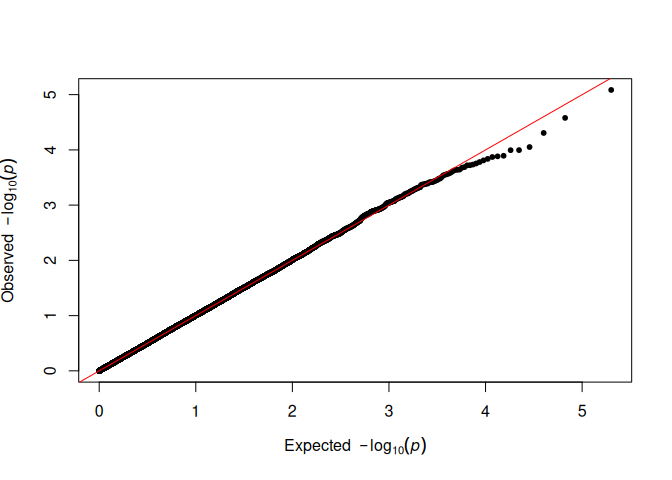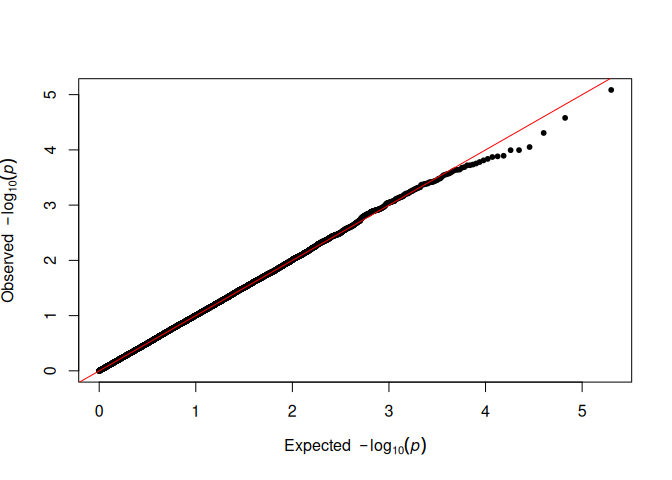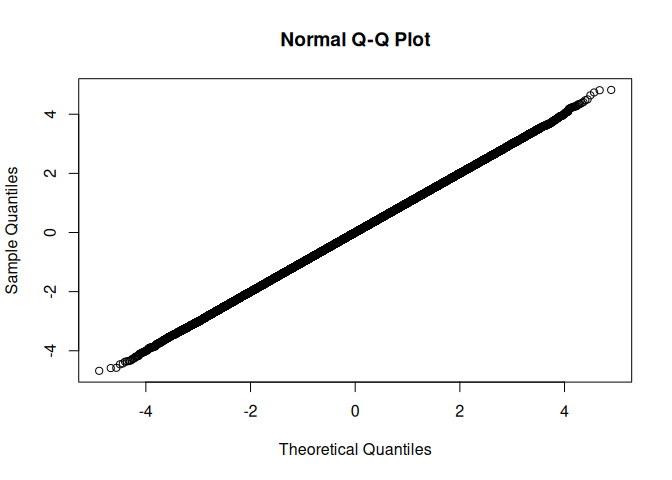# fastqq`fastqq` is intended for creating quantile-quantile plots. We provide faster alternatives to `qqman::qq`, `stats::qqplot` and `stats::qqnorm`. We also provide the function `fastqq::drop_dense` such that the user can extract the data to plot with `ggplot`.

For 100 million samples, we achieve 80X speedup compared to `qqman::qq`. This takes the running time from ~13.5 minutes down to less than 10 seconds.

## Background

This package was originally intended to speedup the creation of QQ plots for genome wide association studies (GWAS). Then I decided to make it a general tool fro QQ style plots. For QQ plots, the user often plots tens to hundreds of millions of points. Creating scatter plots with so many points is usually not efficient since the graphics devices store all the data, such that the visualization can be rescaled or plotted in a vector graphics format (where again all the data is stored).

A better and faster approach in these cases is to note that many of the points are so close to each other that there is no value in including them in the plot. QQ style plots are usually a monotonically increasing sequence of points, so we can easily employ fast filtering to remove redundant points, that would otherwise not be visible in the final plot anyways.

See examples below.

Note that this package is inspired by the `qqman` package, which has now been archived. The interface to the `qq` function should be very similar, and `fastqq::qq` is a drop-in replacement for `qqman::qq`. I created this package since it could take more than 10 minutes to render a single plot with `qqman::qq`. This is also to save on memory and other resources, in particular time.

## Installation

You can install the released version of `fastqq` from CRAN with:

``install.packages("fastqq")``

And the development version from GitHub with:

``````# install.packages("devtools")
devtools::install_github("gumeo/fastqq")``````

## Example

The following is an example from very simple simulated data:

``````suppressPackageStartupMessages(library(fastqq))
set.seed(42)
p_simulated <- runif(1e5)
# Classic way to do this with qqman
qqman::qq(p_simulated)````````````
# Alternative
fastqq::qq(p_simulated)``````There is no visible difference, and the analysis can proceed as usual.

We can compare the timings of creating the plots, with `qqman`.

``````set.seed(555)
N_test <- c(1e3,1e4,1e5,1e6)
time_method <- function(pkg_name, method){
suppressPackageStartupMessages(library(pkg_name,
character.only=TRUE, quietly = TRUE))
for(N in N_test){
p_vec <- runif(n = N)
print(paste0("Timing ", pkg_name, "::", method," with ",
N, " points"))
tictoc::tic()
pdf(file = NULL) # Prevent the plots from appearing
do.call(method, list(pvector=p_vec))
dev.off()
tictoc::toc()
}
}
N_test <- c(1e3,1e4,1e5,1e6,1e8)
time_method('fastqq','qq')
#>  "Timing fastqq::qq with 1000 points"
#> 0.02 sec elapsed
#>  "Timing fastqq::qq with 10000 points"
#> 0.022 sec elapsed
#>  "Timing fastqq::qq with 1e+05 points"
#> 0.024 sec elapsed
#>  "Timing fastqq::qq with 1e+06 points"
#> 0.101 sec elapsed
#>  "Timing fastqq::qq with 1e+08 points"
#> 10.999 sec elapsed
N_test <- c(1e3,1e4,1e5,1e6)
time_method('qqman','qq')
#>  "Timing qqman::qq with 1000 points"
#> 0.003 sec elapsed
#>  "Timing qqman::qq with 10000 points"
#> 0.023 sec elapsed
#>  "Timing qqman::qq with 1e+05 points"
#> 0.218 sec elapsed
#>  "Timing qqman::qq with 1e+06 points"
#> 2.196 sec elapsed``````

So we can expect around 25X speedup for a million points. For 100 million points (order of magnitude for modern GWAS), `fastqq::qq` takes 10 seconds on the same hardware as for the timings above, `qqman::qq` takes more than 13.78 minutes for 100 million points (80X speedup) and if one saves to a vector graphic output, all the data is stored, and the file size scales with the amount of points.

### `qqnorm` example

We can use `qqnorm` just like from `stats::qqnorm`. The only difference is in the output, we return sorted output, and exclude `NA`s.

``````set.seed(42)
suppressPackageStartupMessages(library(fastqq))
fastqq::qqnorm(rnorm(1e6))``````### `qqplot` example

``````set.seed(42)
suppressPackageStartupMessages(library(fastqq))
fastqq::qqplot(rnorm(1e6),rnorm(1e6))``````### `drop_dense` and plot with `ggplot` example

``````set.seed(42)
suppressPackageStartupMessages(library(fastqq))
suppressPackageStartupMessages(library(ggplot2))
x <- rnorm(1e6)
y <- rnorm(1e6)
df <- fastqq::drop_dense(x, y)

ggplot(df, aes(x=x,y=y)) + geom_point()``````# Note on other efforts

After I created this, I have found several sources, that aim at something similar, usually also a manhattan plot (I am probably also missing other packages):

• `fastman` package. Uses `scattermore`, so the plotting is very fast. This package is not currently (31/07/2021) on CRAN.
• `ramwas` package. Has not been maintained in 2 years and is on bioconductor. This package is aimed for Fast Methylome-Wide Association Study Pipeline for Enrichment Platforms and the `ramwas::qqPlotFast` function is just a minor part of the package. It

Here are also some projects on CRAN, where the plotting is similar to `qqman::qq`, and is not improved for speed.